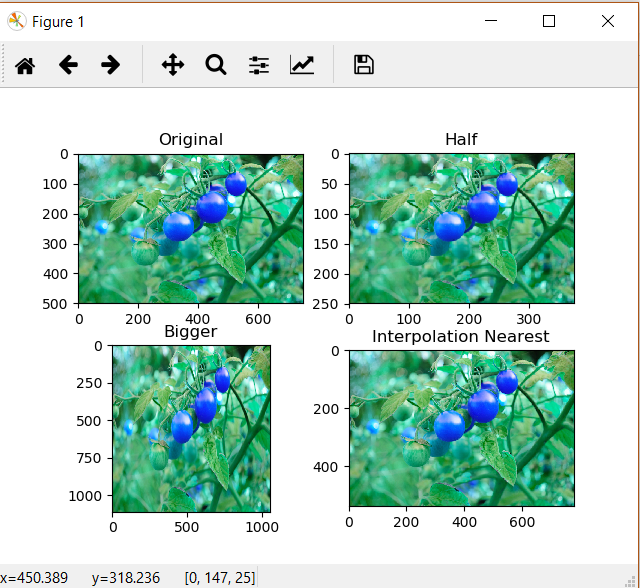# Image Resizing using OpenCV | Python

Image resizing refers to the scaling of images. Scaling comes handy in many image processing as well as machine learning applications. It helps in reducing the number of pixels from an image and that has several advantages e.g. It can reduce the time of training of a neural network as more is the number of pixels in an image more is the number of input nodes that in turn increases the complexity of the model.

It also helps in zooming in images. Many times we need to resize the image i.e. either shirk it or scale up to meet the size requirements. OpenCV provides us several interpolation methods for resizing an image.

Choice of Interpolation Method for Resizing –

• `cv2.INTER_AREA`: This is used when we need need to shrink an image.
• `cv2.INTER_CUBIC`: This is slow but more efficient.
• `cv2.INTER_LINEAR`: This is primarily used when zooming is required. This is the default interpolation technique in OpenCV.

Below is the code for resizing.

 `import` `cv2 ` `import` `numpy as np ` `import` `matplotlib.pyplot as plt  ` `%` `matplotlib qt ` `# This is a magic command to display in an external window ` ` `  `image ``=` `cv2.imread(``"C://gfg//tomatoes.jpg"``, ``1``) ` `# Loading the image ` ` `  `half ``=` `cv2.resize(image, (``0``, ``0``), fx ``=` `0.1``, fy ``=` `0.1``) ` `bigger ``=` `cv2.resize(image, (``1050``, ``1610``)) ` ` `  `stretch_near ``=` `cv2.resize(image, (``780``, ``540``),  ` `               ``interpolation ``=` `cv2.INTER_NEAREST) ` ` `  ` `  `Titles ``=``[``"Original"``, ``"Half"``, ``"Bigger"``, ``"Interpolation Nearest"``] ` `images ``=``[image, half, bigger, stretch_near] ` `count ``=` `4` ` `  `for` `i ``in` `range``(count): ` `    ``plt.subplot(``2``, ``2``, i ``+` `1``) ` `    ``plt.title(Titles[i]) ` `    ``plt.imshow(images[i]) ` ` `  `plt.show() `

Output:Note: One thing to keep in mind while using the `cv2.resize()` function is that the tuple passed for determining the size of new image ((1050, 1610) in this case) follows the order (width, height) unlike as expected (height, width).

My Personal Notes arrow_drop_upCheck out this Author's contributed articles.

If you like GeeksforGeeks and would like to contribute, you can also write an article using contribute.geeksforgeeks.org or mail your article to contribute@geeksforgeeks.org. See your article appearing on the GeeksforGeeks main page and help other Geeks.

Please Improve this article if you find anything incorrect by clicking on the "Improve Article" button below.

Improved By : Sourabh_Sinha, subhamskm52

Article Tags :

2

Please write to us at contribute@geeksforgeeks.org to report any issue with the above content.